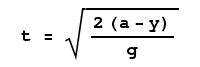Home | Mathematics | * Calculus | * Old Calculus Primer | * Old Calculus Primer 02. A Moving Experience 03. Derivation 04. Integration 05. Volume 1 06. Volume 2 07. Differential Equations 08. Terminal Velocity 09. Nature's Math 10. ToolsShare This Page
Calculus Primer 7: Differential Equations
P. Lutus Message Page

(double-click any word to see its definition)

On this page, we will introduce a new kind of Calculus equation called a differential equation, which is defined as an equation that contains within it a function and one or more derivative terms of that function. As we begin to state this problem, we will need to review the notation used to describe derivatives. Remember from some previous pages that (all with respect to time):

(1)  p(t) = position
(2)  p'(t) = velocity, the first derivative of position
(3)  p''(t) = acceleration, the second derivative of position

We showed earlier that one can derive any of these values in terms of any one the others. For example, if we only have the acceleration derivative, we should be able to obtain velocity and position using it.

Now we can solve an interesting physics problem — the motion of a body falling freely under the influence of gravity. To solve this problem, we need only one physical value, the acceleration produced by gravity:

(4)  g = 9.8 m/s/s (acceleration due to gravity at Earth's surface, meters/second/second)

We can use this physical value as the key expression in these related differential terms:

(5)  p''(t) = -g
(6)  p'(0) = 0
(7)  p(0) = a

This is a typical system of differential equation terms. Term 5 says that the acceleration is equal to g, that is, the acceleration due to gravity, which we have preceded with a minus sign to show that it creates motion in a downward direction. Term 6 says that the velocity at time zero is zero. Term 7 says that the position at time zero is equal to some specified altitude a.

Just as with derivatives and integrals, some differential equations can be solved by writing a new function, while others only allow numerical approximations to be written. And, since this is a Calculus introduction, we will present the following solution without its derivation, in order to avoid losing the general reader who would like to see what Calculus can do without necessarily wanting to fully understand how.

The solution for this example equation is a function y(t) that provides a position y for any time t (we will use y here to avoid confusion with the differential equation terms above):

 (8)  y(t) = a - g t22

This equation tells us our position as a function of time, from a starting altitude a and using a gravitational acceleration g, neglecting air resistance (this will be dealt with in the next page).

One might wonder why we didn't use a specific number instead of g? Well, this allows us to use a different gravitational acceleration, such as exists on the Moon or Mars. As a general rule it is best to delay the application of a specific numerical value as long as possible in systems like this. Now that we have produced a solution for the differential terms listed above expressed as a position with respect to time, we can obtain derivatives for velocity and acceleration that apply to this free-fall equation, and create some useful forms of the equation:

(9)  y'(t) = -g t

Equation 9 tells us that velocity increases linearly with respect to time. Remember, we are neglecting air resistance, a subject for the next page.

All the usual algebraic manipulations apply to differential equations. If we want an equation to take a different form, we can rearrange the terms in the usual way. For example, we can ask how long it might take to fall from a height of 500 meters to the surface, again neglecting air resistance:

 (10)(11)(12)  t = 10.1 seconds

Again, in exchange for being expressed as simple equations, these results suffer from the simplifying assumption that there is no air resistance. Real-world solutions for this problem are not so pretty, but they are more accurate. One is reminded of Einstein's remark, "As far as the laws of mathematics refer to reality, they are not certain, and as far as they are certain, they do not refer to reality."

In the next section we will present a somewhat more complex differential equation that accommodates air resistance.

 Home | Mathematics | * Calculus | * Old Calculus Primer | * Old Calculus Primer 02. A Moving Experience 03. Derivation 04. Integration 05. Volume 1 06. Volume 2 07. Differential Equations 08. Terminal Velocity 09. Nature's Math 10. ToolsShare This Page